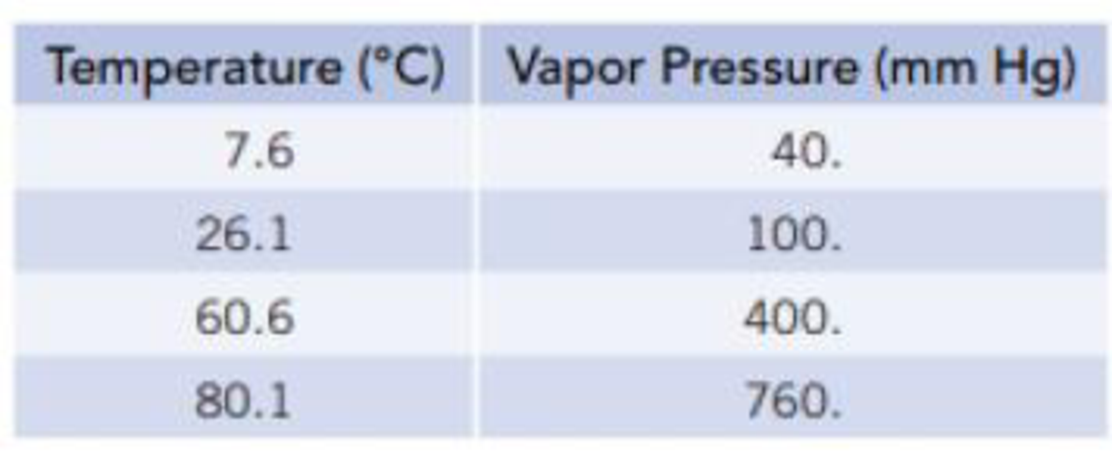Chapter 11, Problem 21PS

Chapter
Section
Textbook Problem

Equilibrium vapor pressures of benzene, C6H6, at various temperatures are given in the table.(a) What is the normal boiling point of benzene? (b) Plot these data so that you have a graph resembling the one in Figure 11.12. At what temperature does the liquid have an equilibrium vapor pressure of 250 mm Hg? At what temperature is the vapor pressure 650 mm Hg? (c) Calculate the molar enthalpy of vaporization for benzene using the Clausius–Clapeyron equation.

(a)

Interpretation Introduction

Interpretation:

The normal boiling point of benzene has to be determined.

Concept Introduction:

Boiling point: It is the temperature at which liquid converts to vapor. At boiling point the vapor pressure of liquid and the pressure of the surroundings are equal.

Normal boiling point: When the external pressure is 760mmHg we can call it as normal boiling point.

Explanation

The normal boiling point of benzene is calculated

Given:

Temperature(K)Vapour Pressure(mmHg)280

(b)

Interpretation Introduction

Interpretation:

The temperature versus vapor pressure graph should be plotted. The temperatures at which the liquid has vapour pressures of 250mmHgand650mmHg has to be determined

Concept Introduction:

Vapor pressure is nothing but the pressure of a vapor in contact with its liquid or solid form.

When a liquid and vapor are in equilibrium the pressure exerted by the vapor is called the equilibrium vapor pressure

(c)

Interpretation Introduction

Interpretation:

The molar enthalpy of vaporization using Clausius-Clapeyron has to be determined

Concept Introduction:

Clausius-Clapeyron equation:

lnP=(ΔvapH0RT)+C

From this relationship we can calculate the molar enthalpy of vaporization by knowing the corresponding temperature and pressure values.

If we have pressures at two different temperatures, then enthalpy of vaporization can be calculated by

lnP2p1=-ΔvapH0R[1T2-1T1]

Still sussing out bartleby?

Check out a sample textbook solution.

See a sample solution

The Solution to Your Study Problems

Bartleby provides explanations to thousands of textbook problems written by our experts, many with advanced degrees!

Get Started